# Revision Notes - Circle Notes | Study Mock Test Series for JEE Main & Advanced 2022 - JEE

## JEE: Revision Notes - Circle Notes | Study Mock Test Series for JEE Main & Advanced 2022 - JEE

The document Revision Notes - Circle Notes | Study Mock Test Series for JEE Main & Advanced 2022 - JEE is a part of the JEE Course Mock Test Series for JEE Main & Advanced 2022.
All you need of JEE at this link: JEE

• The equation of a circle with its center at C(x0, y0) and radius r is: (x – x0)2 + (y – y0)2 = r2
• If x0 = y0 = 0 (i.e. the centre of the circle is at origin) then equation of the circle reduce to x+ y= r2.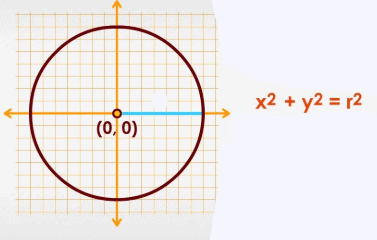• If r = 0 then the circle represents a point or a point circle.
• The equation x2 + y+ 2gx + 2fy + c = 0 is the general equation of a circle with centre (–g, –f) and radius √(g2+f2-c).
• Equation of the circle with points P(x1, y1) and Q(x2, y2) as extremities of a diameter is (x – x1) (x – x2) + (y – y1)(y – y2) = 0.
• For general circle, the equation of the chord is x1x + y1y + g(x1 + x) + f(y1 +y) + c = 0
• For circle x+ y2 = a2, the equation of the chord is x1x + y1y = a2
• The equation of the chord AB
• (A ≡ (R cos α, R sin α); B ≡ (R cos β, R sin β)) of the circle x2 + y= R2 is given by x cos ((α + β )/2) + y sin ((α - β )/2) = a cos ((α - β )/2)
• Chords are equidistant from the center of a circle if and only if they are equal in length.

Equal chords of a circle subtend equal angles at the center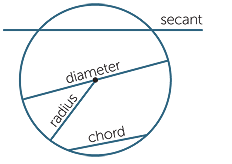• The angle subtended by an arc at the center id double the angle subtended by the same arc at the circumference of the circle.
• Angle between the tangent and the radius is 90°.
• Angles in the same segment are equal.
• Angle in a semi-circle is 90°.
• Two angles at the circumference subtended by the same arc are equal.

The below table describes the equations of circle according to changes in radii and centers: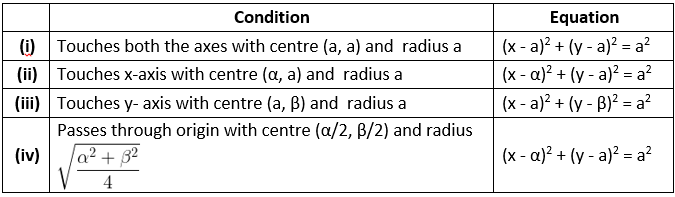• The point P(x1, y1) lies outside, on, or inside a circle?
S ≡ x+ y2 + 2gx + 2fy + c = 0, according as S1 ≡ x12 + y12 + 2gx1 + 2fy+ c > = or < 0.
• The equation of the chord of the circle x+ y2 + 2gx + 2fy +c=0 with M(x1, y1) as the midpoint of the chord is given by:
xx+ yy+ g(x + x1) + f(y + y1) = x1+ y12 + 2gx1 + 2fy1  i.e. T = S1
• In case the radius and the central angle of a triangle are given, the length of the chord can be computed using the formula.
• Length of the chord = 2r sin (c/2), where ‘c’ is the central angle and ‘r’ is the radius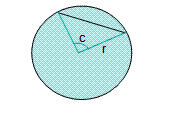• If a circle has two secants QR and ST, then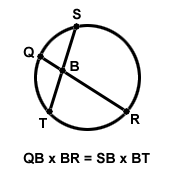• If there is a circle which has one tangent and one secant, then the square of the tangent is equal to the product of the secant segment and its external segment.
• If a radius or the diameter of a circle is perpendicular to a chord, then it divides the chord into two equal parts. The converse also holds true.
Hence, in the below figure, if OB is perpendicular to PQ, then then PA = AQ.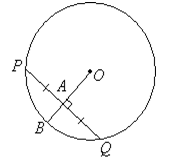• The perpendicular bisector of a chord passes through the center of a circle. In the figure given below, OA is the perpendicular bisector of chord PQ and it passes through the circle. Similarly, OB is the perpendicular bisector of RS and it also passes through center.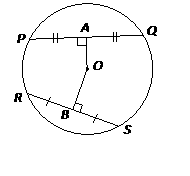• In the above circle, OA is the perpendicular bisector of the chord PQ and it passes through the center of the circle. OB is the perpendicular bisector of the chord RS and it passes through the center of the circle.
• If two chords in a circle are congruent, then they are equidistant from the center.
• If two chords are equidistant from the center, then they are congruent. So, in the figure if PQ = RS then OA = OB and similarly, if OA = OB then PQ = RS.
• The arcs intercepted by two congruent chords in a circle are congruent.
• If two arcs are congruent then their corresponding chords are congruent.
• If a line y = mx + c intersects the circle x2 + y= a2 in two distinct points A and B then length of intercept AB = 2√[(a2(1+m2)-c2)/ (1+m2)].
• The equation of the tangent on a point on the circle and the equations of chord of contact are both represented by T = 0. But the difference between the two is that in case of chord of contact, the point say (x1, y1) lies outside the circle while in case of tangent it lies on the circle.
• Common tangents may be either direct or transverse.
• Direct common tangents to two circles meet on the line joining the centers and hence divide it externally in the ratio of their radii.
• Transverse common tangents also meet on the line of centers as the direct common tangents but the difference being that they divide it internally in the ratio of their radii.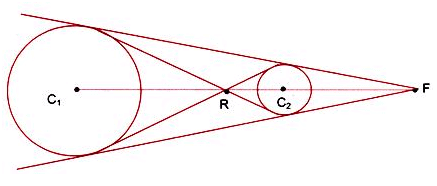• There cannot be any common tangent if a circle lies completely inside another without touching it.
• If two circles touch each other internally one and only one common tangent is possible.
• If two circles touch each other in two distinct points, then in such a case, two common tangents can be drawn.
• In case of two circles touching each other externally, three common tangents can be drawn.
• Four common tangents are possible when two circles neither touch nor intersect but one lies completely inside the other.

How to calculate direct common tangents:

• Suppose there are two circles with centers at C1 and C2 and radii as rand r2 and P is the point of intersection of direct common tangents as shown in the figure below: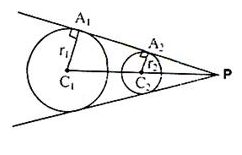C1A1, C2A2 are perpendiculars from C1 and C2 to one of the tangents. The point ‘P’ divides C1Cexternally in the ratio r1: r2. So, in order to find the direct common tangent we just need to find the point P which divides the line joining the center externally in the ratio of radii.
Equation of direct common tangent is SS1 = T2 where S is the equation of one circle.
• Length of C1C2 > |r1 – r2|
• For two circles with equations S and S’, where
S ≡ x+ y+ 2gx + 2fy + c and
S’ ≡ x+ y2 + 2g’x + 2fy’ + c’
Then equation of radical axis of two circles S = 0 and S’ = 0 is given by S = S’
2(g – g’)x + 2(f – f’)y + (c – c’) = 0
• The condition for two circles with centres at C1(x1, y1) and C2(x2, y2) and radii r1, r2 respectively, to touch each other internally and externally a
(a) Internally: If |C1 C2| = |r2 – r1| and the point of contact is ((r1x2-r2x1)/(r1+r2) , (r1y2-r2y1)/(r1+r2)).
(b) Externally: If |C1 C2| = |r2 – r1| and the point of contact is ((r1x2+r2x1)/(r1+r2) , (r1y2+r2y1)/(r1+r2)).
• For the circle S = 0, the equation, S – S’ = 0 represents the equation of the radical axis i.e. the equation of radical axis is
2x(g – g’) + 2y(f – f’) + c – c’ = 0
• If the circles S = 0 and S’ = 0 intersect in real and distinct points, then S – S’ = 0 is the equation of the common chord of the two circles.
• If S’ = 0 and S = 0 touch each other, then S – S’ = 0 is the equation of the common tangent to the two circles at the point of contact.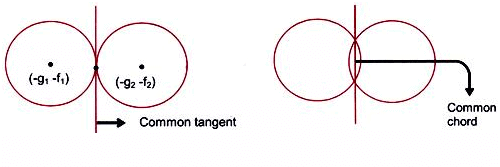The radical axis of two circles is perpendicular to the line joining the centres

• The radical axis of three circles taken two at a time are concurrent and the point of concurrency is known as the radical centre.
• The radical axis of two circles bisects their direct common tangents.
• If two circles cut a third circle orthogonally, then the radical axis of the two circles will pass through the centre of the third circle.
• If in a system of circles, every two of them have the same radical axis, then the system is a coaxal system

The equations of family of circles in various cases:

• S+ λS2 = 0 is the family of circles passing through the intersection of S1 = 0 and S2 = 0.
• The family of circles passing through the intersection of line L and circle S is given by S + λL = 0.  A particular value of λ in the equation yields a unique circle.
• Family of circles passing through two given points A(x1, y1) and B(x2, y2) are given by the equation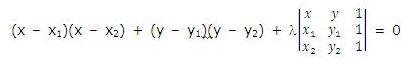• Two circles S = 0 and S1 = 0 with centres at (–g, –f) and (–g1, –f1) cut each other orthogonally if the following equation is satisfied:
• 2gg1+ 2ff= c + c

The document Revision Notes - Circle Notes | Study Mock Test Series for JEE Main & Advanced 2022 - JEE is a part of the JEE Course Mock Test Series for JEE Main & Advanced 2022.
All you need of JEE at this link: JEEUse Code STAYHOME200 and get INR 200 additional OFF

## Mock Test Series for JEE Main & Advanced 2022

2 videos|258 docs|160 tests

Track your progress, build streaks, highlight & save important lessons and more!

,

,

,

,

,

,

,

,

,

,

,

,

,

,

,

,

,

,

,

,

,

;